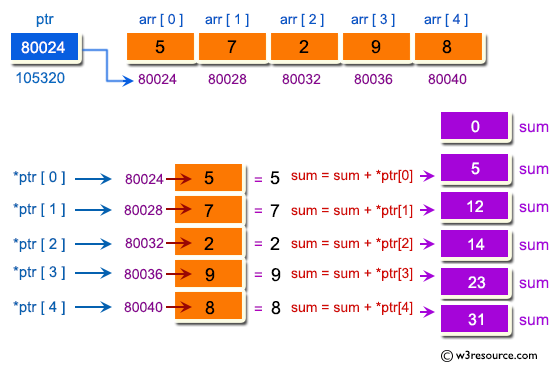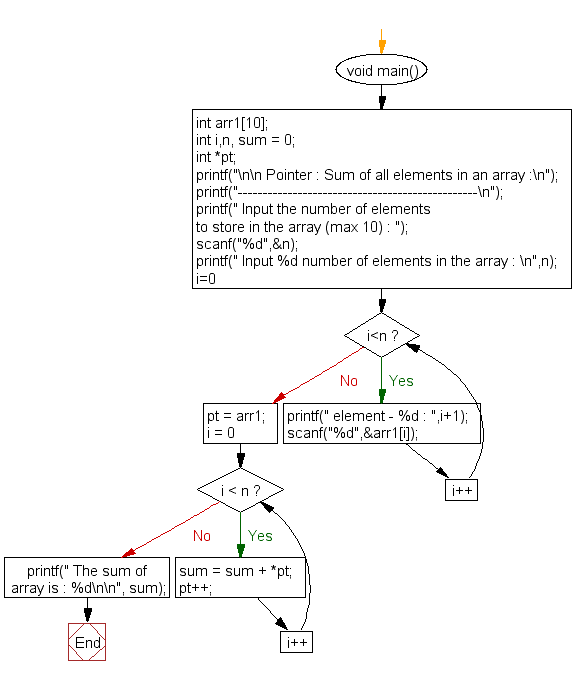﻿ C Program: Sum of all elements in an array - w3resource# C Exercises: Sum of all elements in an array

## C Pointer : Exercise-16 with Solution

Write a program in C to compute the sum of all elements in an array using pointers.

Pictorial Presentation:Sample Solution:

C Code:

``````#include <stdio.h>
void main()
{
int arr1;
int i,n, sum = 0;
int *pt;

printf("\n\n Pointer : Sum of all elements in an array :\n");
printf("------------------------------------------------\n");

printf(" Input the number of elements to store in the array (max 10) : ");
scanf("%d",&n);

printf(" Input %d number of elements in the array : \n",n);
for(i=0;i<n;i++)
{
printf(" element - %d : ",i+1);
scanf("%d",&arr1[i]);
}

pt = arr1; // pt store the base address of array arr1

for (i = 0; i < n; i++) {
sum = sum + *pt;
pt++;
}

printf(" The sum of array is : %d\n\n", sum);
}
```
```

Sample Output:

``` Pointer : Sum of all elements in an array :
------------------------------------------------
Input the number of elements to store in the array (max 10) : 5
Input 5 number of elements in the array :
element - 1 : 2
element - 2 : 3
element - 3 : 4
element - 4 : 5
element - 5 : 6
The sum of array is : 20
```

Flowchart:C Programming Code Editor:

Have another way to solve this solution? Contribute your code (and comments) through Disqus.

What is the difficulty level of this exercise?

Test your Programming skills with w3resource's quiz.

﻿

## C Programming: Tips of the Day

How to format strings using printf() to get equal length in the output?

You can specify a width on string fields, e.g.

`printf("%-20s", "initialization..."); `

And then whatever's printed with that field will be blank-padded to the width you indicate.

The - left-justifies your text in that field.

Ref : https://bit.ly/34DMOc3